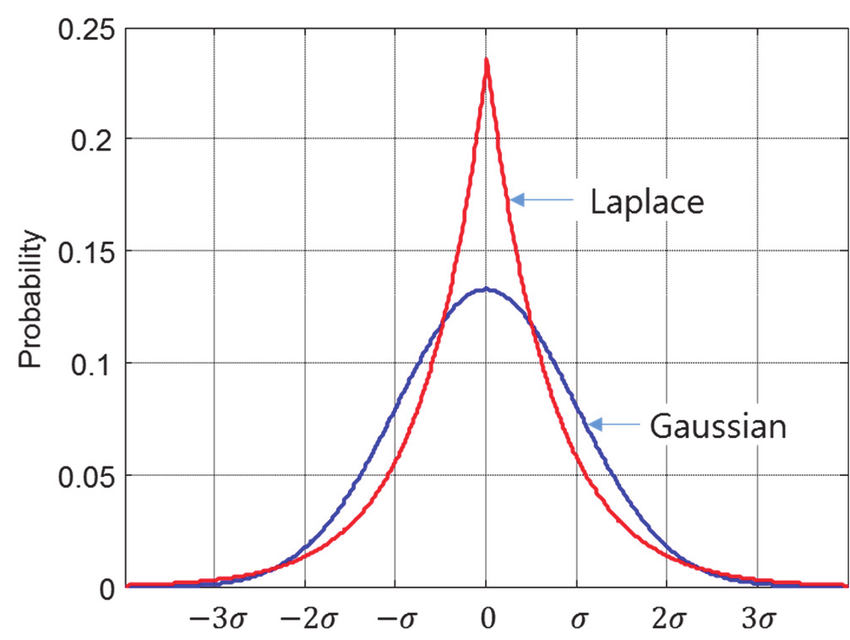# 剖析深度學習 (3)：MLE、MAP差在哪？談機器學習裡的兩大統計觀點

Posted on March 07, 2020 in AI.ML. View: 17,587

### 條件機率

• 邊際機率（marginal probability）

$$p(X=x_i)=\sum_j p(X=x_i, Y=y_j) \ \ ↪︎【1】$$
(如下圖所示)

• 條件機率（conditional probability）

$$p(X=x_i, Y=y_j)=p(Y=y_j\mid X=x_i)p(X=x_i) \ \ ↪︎【2】$$
(如下圖所示)

• 條件機率的鏈鎖法則

$$p(a,b,c)=p(a\mid b,c)\cdot p(b,c)=p(a\mid b,c)\cdot p(b\mid c)\cdot p(c) \ \ ↪︎【3】$$
(因為 $$a\cap b\cap c=a\cap (b\cap c)$$)

• 獨立性

• if X and Y are independent, $$p(X=x_i,Y=y_j)=p(X=x_i)\cdot p(Y=y_j)$$

• if X and Y are independent, $$p(X=x_i,Y=y_j\mid Z=z_k)=p(X=x_i\mid Z=z_k)\cdot p(Y=y_j\mid Z=z_k) \ \ ↪︎【4】$$### Maximum Likelihood Estimation (MLE)

$$\theta_{MLE}=argmax_\theta\ p(\mathcal{D}\mid m,\theta) \ \ ↪︎【5】$$

$$=argmax_\theta\ p_{\mathcal{D}_1,...,\mathcal{D}_N}(d_1,...,d_N\mid m,\theta) \ \ ↪︎【6】$$

$$=argmax_\theta\ \prod_{i}\ p_{\mathcal{D}_i}(d_i\mid m,\theta) \ \ ↪︎【7】$$

$$\theta_{MLE}=argmax_\theta\ \prod_{i}\ p(d_i\mid m,\theta) \ \ ↪︎【8】$$

$$\theta_{MLE}=argmax_\theta\ \sum_{i}\ \operatorname{ln}p(d_i\mid m,\theta) \ \ ↪︎【9】$$

$$\theta_{MLE}=argmax_\theta\ \sum_{i}\ \operatorname{ln}p(x_i,y_i\mid m,\theta) \ \ ↪︎【10】$$

$$=argmax_\theta\ \sum_{i}\ \operatorname{ln}[p(y_i\mid x_i,m,\theta)p(x_i\mid m,\theta)] \ \ ↪︎【11】$$

$$x_i$$ 與 model參數 $$m$$$$\theta$$ 應該是互相獨立的，

$$=argmax_\theta\ \sum_{i}\ \operatorname{ln}[p(y_i\mid x_i,m,\theta)p(x_i)] \ \ ↪︎【12】$$
$$=argmax_\theta\ \sum_{i}\ \operatorname{ln}p(y_i\mid x_i,m,\theta)+\operatorname{ln}p(x_i) \ \ ↪︎【13】$$

$$p(x_i)$$$$\theta$$ 無關，在優化過程可忽略，得： ⚠️

$$\theta_{MLE}=argmax_\theta\ \sum_{i}\ \operatorname{ln}p(y_i\mid x_i,m,\theta) \ \ ↪︎【14】$$

$$\theta_{MLE}=argmin_\theta\ \frac{1}{N}\sum_{i}-\operatorname{ln}p(y_i\mid x_i,m,\theta) \ \ ↪︎【15】$$

$$\theta_{MLE}=argmin_\theta\ E_{x\sim p_{data}}[-\operatorname{ln}p_{model}(y_i\mid x_i,m,\theta)]＝argmin_\theta\ H(p_{data},p_{model}) \ \ ↪︎【16】$$

$$D_{KL}(p_{data}\| p_{model})=E_{x\sim p_{data}}[\operatorname{ln}p_{data}(x)-\operatorname{ln}p_{model}(x)] \ \ ↪︎【17】$$

### Maximum A Posterior (MAP)

• 第一組： $$\theta_1=0.5,\ \theta_2=0.1,\ \theta_3=-0.1$$
• 第二組： $$\theta_1=1000.0,\ \theta_2=12.5,\ \theta_3=-500.0$$

$$p(\mathcal{D}, m, \theta)=p(\mathcal{D},m\mid \theta)p(\theta)=p(\theta\mid \mathcal{D},m)p(\mathcal{D},m) \ \ ↪︎【18】$$

$$p(\theta\mid \mathcal{D},m)=\frac{p(\mathcal{D},m\mid \theta)p(\theta)}{p(\mathcal{D},m)} \ \ ↪︎【19】$$

$$\frac{p(\mathcal{D},m\mid \theta)p(\theta)}{p(\mathcal{D},m)}=\frac{p(\mathcal{D}\mid m,\theta)[p(m\mid \theta)p(\theta)]}{p(\mathcal{D\mid m})p(m)}＝\frac{p(\mathcal{D}\mid m,\theta)}{p(\mathcal{D}\mid m)}\frac{p(m,\theta)}{p(m)}=\frac{p(\mathcal{D}\mid m,\theta)p(\theta\mid m)}{p(\mathcal{D}\mid m)} \ \ ↪︎【20】$$

$$p(\theta\mid \mathcal{D},m)=\frac{p(\mathcal{D}\mid m,\theta)p(\theta\mid m)}{p(\mathcal{D}\mid m)} \ \ ↪︎【21】$$

• $$p(\mathcal{D}\mid m,\theta)$$ 就是Likelihood，Maximum這一項就是MLE，不用再特別討論。
• $$p(\theta\mid m)$$ 稱為先驗機率 (Prior Probability)，它所代表的意義正是描述 $$\theta$$ 這個參數在給定 $$m$$ 之後出現的機率有多大，這一項是先於經驗的，這裡的經驗指的是「這一次的實驗」，而這個 $$p(\theta\mid m)$$ 是與本次實驗 $$\mathcal{D}$$ 無關的。因此這個 $$p(\theta\mid m)$$ 是需要人為給定的，你可以自己假設分布，例如：假設為有最少假設的Normal Distribution，或者是從過去的歷史紀錄去統計出 $$p(\theta\mid m)$$ 也行。
• $$p(\mathcal{D}\mid m)$$ 稱為資料機率（probability of data），通常數據與模型的Hyperparameters應該是相互獨立的，所以其實可以簡寫成 $$p(\mathcal{D})$$ ，這一項只需要統計一下Dataset的分布即可得到。
• $$p(\theta\mid \mathcal{D},m)$$ 稱為後驗機率 (Posterior Probability)，它所代表的意義是給定數據 $$\mathcal{D}$$ 和 Hyperparameters $$m$$ 之後，會出現 $$\theta$$ 的機率，有注意到嗎？我們在【21】中同時連結了先驗機率和後驗機率，這代表的是手上原先有一個 $$\theta$$ 分布（先驗機率），經過觀察數據後，我重新的去更新這個 $$\theta$$ 分布（後驗機率），這充分的傳達了貝氏學派的演進概念。

$$\theta_{MAP}=argmax_\theta\ p(\theta\mid \mathcal{D},m)=argmax_\theta\ \frac{p(\mathcal{D}\mid m,\theta)p(\theta\mid m)}{p(\mathcal{D}\mid m)} \ \ ↪︎【22】$$

$$\theta_{MAP}=argmax_\theta\ [\prod_{i}\ \frac{p(d_i\mid m,\theta)}{p(d_i\mid m)}]p(\theta\mid m) \ \ ↪︎【23】$$

$$\theta_{MAP}=argmax_\theta\ \{\sum_{i}\ [\operatorname{ln}p(d_i\mid m,\theta)-\operatorname{ln}p(d_i\mid m)]\}+\operatorname{ln}p(\theta\mid m) \ \ ↪︎【24】$$

$$\theta_{MAP}=argmax_\theta\ \{\sum_{i}\ \operatorname{ln}p(d_i\mid m,\theta)\}+\operatorname{ln}p(\theta\mid m) \ \ ↪︎【25】$$

$$\theta_{MAP}=argmax_\theta\ \{\sum_{i}\ \operatorname{ln}p(y_i\mid x_i,m,\theta)\}+\operatorname{ln}p(\theta\mid m) \ \ ↪︎【26】$$

• 假設 $$p(\theta\mid m)$$ 為一個Uniform Distribution，也就是說所有的 $$\theta$$ 出現機率均等，則 $$p(\theta\mid m)=const.$$，這麼一來這一項在【26】式可以直接槓掉，因為它與 $$\theta$$ 無關，此時 $$\theta_{MAP}=\theta_{MLE}$$ 。所以說在貝氏學派的觀點下，MLE只是MAP的一個特例，MLE只是假設 $$\theta$$ 出現機率均等的MAP，上一講有提過Uniform Distribution為所有分布當中Entropy最大的，也就是不確定程度最大的，也就是人為假設幾乎為零的分布，確實符合頻率學派的觀點：不需要太多人為假設。

• 假設 $$p(\theta\mid m)$$ 為一個Normal Distribution且平均值為0，則
$$p(\theta\mid m)=\frac{1}{\sqrt{2\pi}\sigma}exp\{{-\frac{\theta^2}{2\sigma^2}}\} \ \ ↪︎【27】$$
$$\operatorname{ln}p(\theta\mid m)=\operatorname{ln}(\frac{1}{\sqrt{2\pi}\sigma})-\frac{\theta^2}{2\sigma^2} \ \ ↪︎【28】$$

$$\theta_{MAP}=argmin_\theta\ \sum_{i}-\operatorname{ln}p(y_i\mid x_i,m,\theta)+\frac{1}{2\sigma^2}\theta^2 \ \ ↪︎【29】$$

• 假設 $$p(\theta\mid m)$$ 為一個Laplace Distribution且平均值為0，則
$$p(\theta\mid m)=\frac{1}{2b}exp\{{-\frac{|\theta|}{b}}\} \ \ ↪︎【30】$$
$$\operatorname{ln}p(\theta\mid m)=\operatorname{ln}(\frac{1}{2b})-\frac{|\theta|}{b} \ \ ↪︎【31】$$

$$\theta_{MAP}=argmin_\theta\ \sum_{i}-\operatorname{ln}p(y_i\mid x_i,m,\theta)+\frac{1}{b}|\theta| \ \ ↪︎【32】$$Courtesy Youngjoo Kim

### 結語

$$\theta_{MLE}=argmax_\theta\ \sum_{i}\ \operatorname{ln}p(y_i\mid x_i,m,\theta)$$

$$\theta_{MAP}=argmax_\theta\ \sum_{i}\ \operatorname{ln}p(y_i\mid x_i,m,\theta)+\operatorname{ln}p(\theta\mid m)$$

### Reference

[此文章為原創文章，轉載前請註明文章來源]